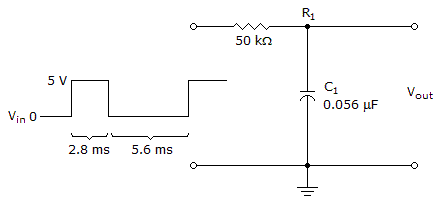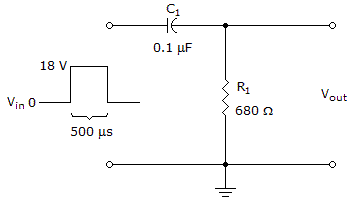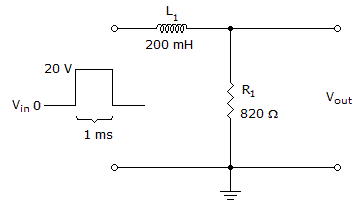# Electronics - Time Response of Reactive Circuits

### Exercise :: Time Response of Reactive Circuits - Filling the Blanks

6.The voltage across the capacitor in the given circuit is _______ at the beginning of the second pulse. Assume the capacitor is initially uncharged.

 A. 0 V B. 427.74 mV C. 2.8 V D. 7.56 V

Explanation:

No answer description available for this question. Let us discuss.

7.If the pulse width were cut in half in the given circuit, the voltage across the resistor would be ______ at the end of the pulse.

 A. 0 V B. 0.36 V C. 0.46 V D. 0.9 V

Explanation:

No answer description available for this question. Let us discuss.

8.If the pulse source has an internal resistance of 80in the given circuit, it will take ______ for the output voltage to decrease to zero.

 A. 1 ms B. 1.1 ms C. 1.2 ms D. 2.5 ms

Explanation:

No answer description available for this question. Let us discuss.

9.

A(n) _____ will decrease the time constant in an RC integrator or differentiator.

 A. open resistor B. open capacitor C. shorted capacitor D. leaky capacitor

Explanation:

No answer description available for this question. Let us discuss.

10.If the component positions were switched in the given circuit, the circuit would be an ______.

 A. RL differentiator B. RC differentiator C. RL integrator D. RC integrator